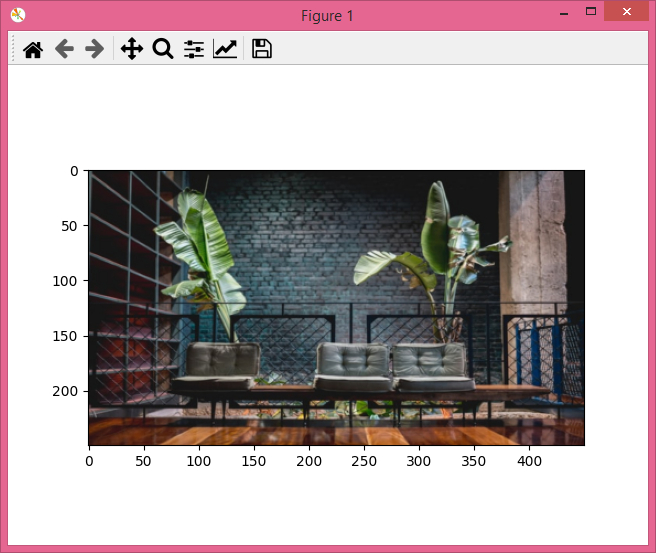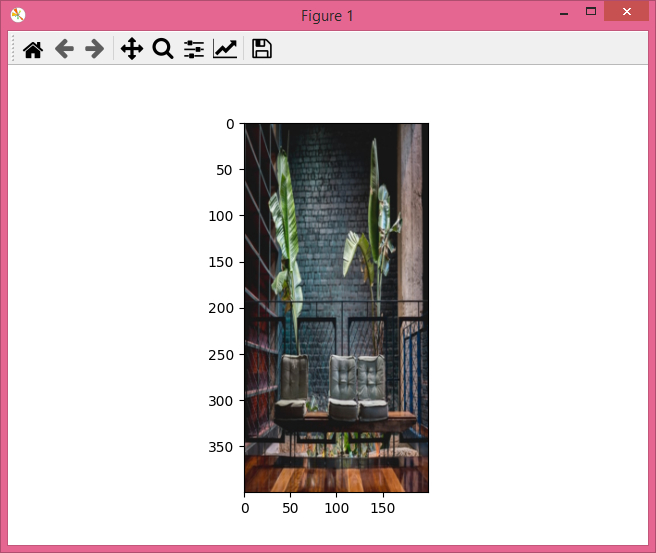# PyTorch â€“ How to resize an image to a given size?

The Resize() transform resizes the input image to a given size. It's one of the transforms provided by the torchvision.transforms module. Resize() accepts both PIL and tensor images. A tensor image is a torch tensor with shape [C, H, W], where C is the number of channels, H is the image height, and W is the image width.

This transform also accepts a batch of tensor images, which is a tensor with [B, C, H, W] where B is the number of images in the batch. If the image is neither a PIL image nor a tensor image, then we first convert it to a tensor image and then apply the Resize()transform.

## Syntax

torchvision.transforms.Resize(size)(img)

• Size – Size to which the input image is to be resized. size is a sequence like (h, w), where h and w are the height and width of the output image. If size is an int, then the resized image will be a square image.

It returns a resized image of given size.

## Steps

We could use the following steps to resize an input image to a given size.

• Import the required libraries. In all the following examples, the required Python libraries are torch, Pillow, and torchvision. Make sure you have already installed them.

import torch
import torchvision
import torchvision.transforms as T
from PIL import Image
import matplotlib.pyplot as plt
• Read the input image. The input image is a PIL image or a torch tensor or a batch of torch tensors.

img = Image.open('lounge.jpg')

• Define a transform to resize the image to a given size. For example, the given size is (300,350) for rectangular crop and 250 for square crop. Change the crop size according your need.

# transform for rectangular resize
transform = T.Resize((300,350))

# transform for square resize
transform = T.Resize(250)
• Apply the above-defined transform on the input image to resize the input image.

resized_img = transform(img)

• Show the resized image.

plt.imshow(resized_img)

## Input Image

This image is used as the input file in all the following examples.## Example 1

The input image is resized to (300, 350). The original image is of size (700,700)

# import the required libraries
import torch
import torchvision.transforms as T
from PIL import Image
import matplotlib.pyplot as plt

img = Image.open('lounge.jpg')

# compute the size(width, height) of image
size = img.size
print("Size of the Original image:", size)

# define transformt o resize the image with given size
transform = T.Resize(size = (250,450))

# apply the transform on the input image
img = transform(img)
print("Size after resize:", img.size)
plt.imshow(img)
plt.show()

## Output

It will return the resized image and also print the size of the original image and the output image on the console.

Size of the Original image: (640, 427)
Size after resize: (450, 250)## Example 2

Let's take another example −

import torch
import torchvision.transforms as T
from PIL import Image
import matplotlib.pyplot as plt

img = Image.open('lounge.jpg')
size = img.size
print("Size of Original image:", size)

transform = T.Resize(size = (400,200))
img = transform(img)

plt.imshow(img)
print("Size after resize:", img.size)
plt.show()

## Output

It will produce the following output −Size of the Original image: (640, 427)
Size after resize: (200, 400)

Updated on: 06-Jan-2022

22K+ Views